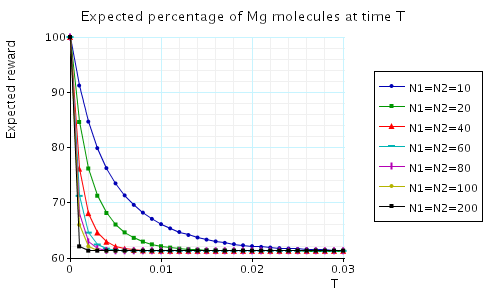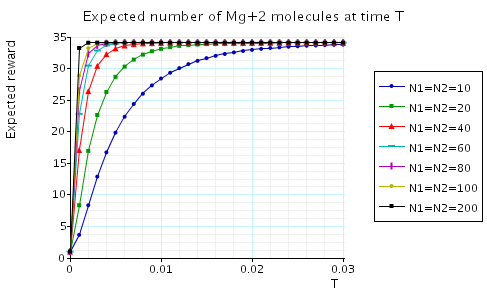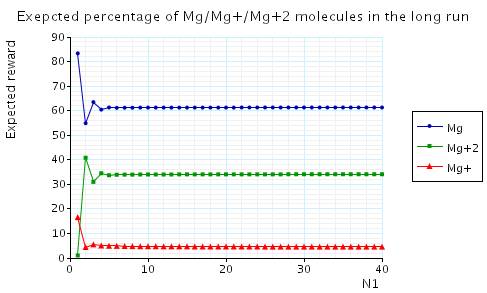# Simple Molecular Reactions

(Shapiro)

### Introduction

In this case study we consider a number of molecular reactions taken from Ehud Shapiro's lecture notes on Biomolecular Processes as Concurrent Computation.

In this approach, the time until a reaction between two molecules is modelled as an exponential distribution, and therefore we construct a CTMC model of the system where the states of the CTMC correspond to the number of molecules of each type and the transitions correspond to the possible reactions between the molecules. The rate of a reaction is determined by a base rate and the concentrations of the reactants (i.e. the number of each type of molecule that takes part in the reaction).

Note that, for each of the chemical reactions we consider, the rate of the reaction is the base rate multiplied by the product of the number of molecules of each type that take part in the reaction. Therefore, to model this is PRISM, we use the fact that in PRISM the rate of synchronous transition is defined as the product of the rates of the local transitions which form this synchronous transition.

### The reaction Na + Cl ↔ Na+ + Cl-

The PRISM source code for this reaction is given below.

```// Na + Cl <--> Na+ + Cl-
// dxp/gxn 09/06/04

ctmc

// constants
const int N1; // number of Na molecules
const int N2; // number of Cl molecules

// Na and Na+ module
module na

na : [0..N1] init N1;
// total number of Na and Na+ molecules is fixed at N1
// na is the number of Na molecules
// therefore N1-na gives the number of Na+ molecules

[e1] na>0  ->     na  : (na'=na-1);
[e2] na<N1 -> (N1-na) : (na'=na+1);

endmodule

// Cl and Cl- module
module cl

cl : [0..N2] init N2;
// total number of Cl and Cl- molecules is fixed at N2
// cl is the number of Cl molecules
// therefore N2-cl is the number of Cl- molecules

[e1] cl>0  ->     cl  : (cl'=cl-1);
[e2] cl<N2 -> (N2-cl) : (cl'=cl+1);

endmodule

// base rates
const double e1rate = 100; // Na + Cl -> Na+ + Cl-
const double e2rate = 10; // Na+ + Cl- -> Na + Cl

// module representing the base rates of reactions
module base_rates

[e1] true -> e1rate : true;
[e2] true -> e2rate : true;

endmodule

// rewards: "percentage of Na molecules present (out of all Na/Na+ molecules)"
rewards "percentage_na"
true : 100*na/N1;
endrewards
```

The table below shows statistics for the CTMCs we have built for different numbers values of the constants N1 and N2. The tables include:

• the number of states and transitions in the CTMC representing the model;
• both the number of nodes and leaves of the MTBDD which represents the model;
• the construction time which equals the time taken for the system description to be parsed and converted to the MTBDD representing it, and the time to perform reachability, identify the non-reachable states and filter the MTBDD accordingly;
• the number of iterations required to find the reachable states (which is performed via a BDD based fixpoint algorithm).
 N1: N2: Model: MTBDD: Construction: States: Transitions: Nodes: Leaves: Time (s): Iter.s: 10 10 11 20 247 21 0.061 11 20 20 21 40 577 41 0.065 21 40 40 41 80 1,317 81 0.104 41 60 60 61 120 1,967 81 0.159 61 80 80 81 160 2,957 161 0.280 81 100 100 101 200 3,695 201 0.351 101 250 250 251 500 10,231 501 2.742 251

Properties:

• Probability of there being i Na molecules at time T?
• Expected percentage of Na molecules at time T?
• Expected long-run percentage of Na molecules?

These properties are expressed in PRISM as follows.

```// Constants:
const double T; // time instant
const int i; // number of molecules

// Probability of there being i Na molecules at time T?
P=? [ true U[T,T] na=i ]

// Expected percentage of Na molecules at time T?
R=? [ I=T ]

// Expected long-run percentage of Na molecules?
R=? [ S ]
```
• The following graph plots, in the case when N1=N2=10, the probability of there being i Na molecules at time as the value of T varies.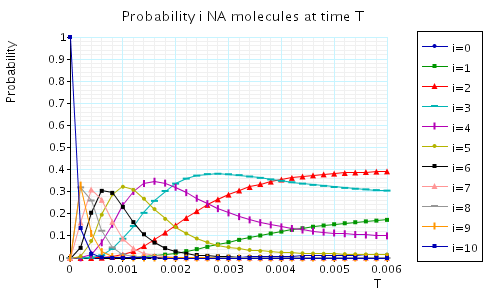• In the graph below we have plotted the expected values percentage of Na molecules at time T as T varies.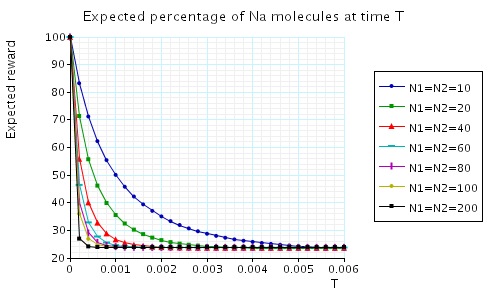• In the graph below we have plotted the expected long-run percentage of Na molecules as N1 varies.### The reaction K + Na + 2Cl ↔ K+ + Na- + 2Cl-

The PRISM source code for this reaction is given below.

```// K + Na + 2Cl <--> K+ + Na+ + 2Cl-
// dxp/gxn 04/10/04

ctmc

// constants
const int N1; // number of Na molecules
const int N2; // number of Cl molecules
const int N3; // number of K molecules

// Na and Na+ module
module na

na : [0..N1] init N1;
// total number of Na and Na+ molecules is fixed at N1
// na is the number of Na molecules
// therefore N1-na gives the number of Na+ molecules

[e1] na>0  ->     na  : (na'=na-1);
[e2] na<N1 -> (N1-na) : (na'=na+1);

endmodule

// Cl and Cl- module
module cl

cl : [0..N2] init N2;
// total number of Cl and Cl- molecules is fixed at N2
// cl is the number of Cl molecules
// therefore N2-cl is the number of Cl- molecules

// reactions with Cl
[e1] cl>0  ->     cl  : (cl'=cl-1);
[e3] cl>0  ->     cl  : (cl'=cl-1);
// reactions with CL-
[e2] cl<N2 -> (N2-cl) : (cl'=cl+1);
[e4] cl<N2 -> (N2-cl) : (cl'=cl+1);

endmodule

// K and K+ module
module k

k : [0..N3] init N3;
// total number of K and K+ molecules is fixed at N3
// k is the number of K molecules
// therefore N3-k gives the number of K+ molecules

[e3] k>0  ->     k  : (k'=k-1);
[e4] k<N3 -> (N3-k) : (k'=k+1);

endmodule

// base rates
const double e1rate = 100; // Na + Cl  -> Na+ + Cl-
const double e2rate = 10;  // Na+ + Cl- -> Na + Cl
const double e3rate = 30;  // K + Cl  -> K+ + Cl-
const double e4rate = 20;  // K+ + Cl- -> K + Cl

// module representing the base rates of reactions
module base_rates

dummy : bool; // dummy variable

[e1] true -> e1rate : true;
[e2] true -> e2rate : true;
[e3] true -> e3rate : true;
[e4] true -> e4rate : true;

endmodule

// reward structure (percentage of Na)
rewards "percentage_na"
true : 100*na/N1;
endrewards

// reward structure (percentage of K)
rewards "percentage_k"
true : 100*k/N3;
endrewards
```

The table below shows statistics for the CTMCs we have built for different numbers values of the constants N1 and N2. The tables include:

• the number of states and transitions in the CTMC representing the model;
• both the number of nodes and leaves of the MTBDD which represents the model;
• the construction time which equals the time taken for the system description to be parsed and converted to the MTBDD representing it, and the time to perform reachability, identify the non-reachable states and filter the MTBDD accordingly;
• the number of iterations required to find the reachable states (which is performed via a BDD based fixpoint algorithm).
 N1: N2: N3: Model: MTBDD: Construction: States: Transitions: Nodes: Leaves: Time (s): Iter.s: 10 10 10 66 220 2,554 93 0.073 11 20 20 20 231 840 11,200 357 0.15 21 40 40 40 861 3,280 48,830 1,192 0.512 41 60 60 40 1,891 7,320 107,376 2,615 1.262 61 80 80 80 3,321 12,960 215,205 4,583 2.232 81 100 100 100 5,151 20,200 332,351 6,821 3.618 101 250 250 250 31,626 125,500 2,272,315 40,105 26.44 251

Properties:

• Probability of there being i Na/K molecules at time T?
• Expected percentage of Na/K molecules at time T?
• Expected long-run percentage of Na/K molecules?

These properties are expressed in PRISM as follows.

```// Constants:
const double T; // time instant
const int i; // number of molecules

// Probability of there being i Na/K molecules at time T?
P=? [ true U[T,T] na=i ]
P=? [ true U[T,T] k=i ]

// Expected percentage of Na/K molecules at time T?
R{"percentage_na"}=? [ I=T ]
R{"percentage_k"}=? [ I=T ]

// Expected long-run percentage of Na/K molecules?
R{"percentage_na"}=? [ S ]
R{"percentage_k"}=? [ S ]
```
• The following graph plots, in the case when N1=N2=N3=10, the probability of there being i Na/K molecules at time T as the value of T varies.• In the graph below we have plotted the expected percentage of Na/K molecules at time T as T varies.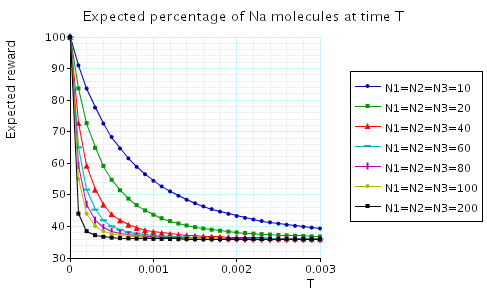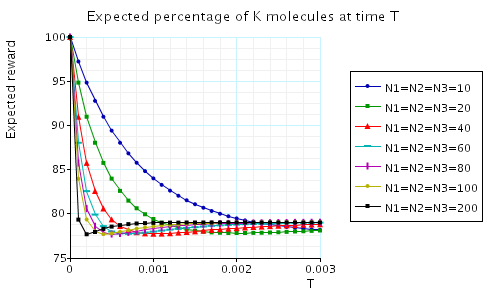• In the graph below we have plotted the expected long-run percentage of Na/K molecules as N1 varies.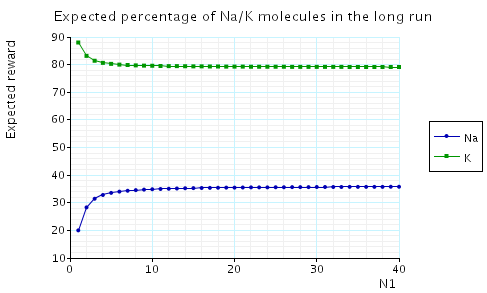### The reaction Mg + 2Cl ↔ Mg+2 + 2Cl-

The PRISM source code for this reaction is given below.

```// Mg + 2Cl <--> Mg+2 + 2Cl-
// dxp/gxn 04/10/04

ctmc

// constants
const int N1; // number of Mg molecules
const int N2; // number of Cl molecules

// Cl and Cl- module
module cl

cl : [0..N2] init N2;
// total number of Cl and Cl- molecules is fixed at N2
// cl is the number of Cl molecules
// therefore N2-cl is the number of Cl- molecules

// reactions with Cl
[e1] cl>0  ->     cl  : (cl'=cl-1);
[e2] cl>0  ->     cl  : (cl'=cl-1);
// reactions with CL-
[e3] cl<N2 -> (N2-cl) : (cl'=cl+1);
[e4] cl<N2 -> (N2-cl) : (cl'=cl+1);

endmodule

// Mg, Mg+ and Mg+2 module
module mg

mg   : [0..N1] init N1;
mg_p : [0..N1] init 0;
// total number of Mg and Mg+ and Mg+2 molecules is fixed at N1
// mg is the number of Mg molecules
// mg_p is the number of Mg molecules
// therefore N1-(mg+mg+) gives the number of Mg+2 molecules

[e1] mg>0 & mg_p<N1 -> mg   : (mg'=mg-1) & (mg_p'=mg_p+1);
[e2] mg_p>0         -> mg_p : (mg_p'=mg_p-1);
[e3] mg_p>0 & mg<N1 -> mg_p : (mg'=mg+1) & (mg_p'=mg_p-1);
[e4] mg_p+mg<N1     -> N1-(mg_p+mg) : (mg_p'=mg_p+1);

endmodule

// base rates
const double e1rate = 10;  // Mg   + Cl  --> Mg+ + Cl-
const double e2rate = 100; // Mg+  + Cl  --> Mg+2 + Cl-
const double e3rate = 50;  // Mg+  + Cl- --> Mg + Cl
const double e4rate = 5;   // Mg+2 + Cl- --> Mg+ + Cl-

// module representing the base rates of reactions
module base_rates

dummy : bool; // dummy variable

[e1] true -> e1rate : true;
[e2] true -> e2rate : true;
[e3] true -> e3rate : true;
[e4] true -> e4rate : true;

endmodule

// reward structur: percentage of Mg molecules
rewards "percentage_mg"
true : 100*mg/N1;
endrewards

// reward structur: percentage of Mg+ molecules
rewards "percentage_mgplus"
true : 100*mg_p/N1;
endrewards

// reward structur: percentage of Mg+2 molecules
rewards "percentage_mgplus2"
true : max(100*(N1-(mg_p+mg))/N1,0);
endrewards
```

The table below shows statistics for the CTMCs we have built for different numbers values of the constants N1 and N2. The tables include:

• the number of states and transitions in the CTMC representing the model;
• both the number of nodes and leaves of the MTBDD which represents the model;
• the construction time which equals the time taken for the system description to be parsed and converted to the MTBDD representing it, and the time to perform reachability, identify the non-reachable states and filter the MTBDD accordingly;
• the number of iterations required to find the reachable states (which is performed via a BDD based fixpoint algorithm).
 N1: N2: Model: MTBDD: Construction: States: Transitions: Nodes: Leaves: Time (s): Iter.s: 10 10 36 110 1,214 64 0.070 11 20 20 121 420 5,110 224 0.123 21 40 40 441 1,640 21,727 765 0.402 41 60 60 961 3,660 47,064 1,630 0.798 61 80 80 1,681 6,480 94,413 2,818 1.623 81 100 100 2,601 10,100 145,234 4,262 2.536 101 250 250 15,876 62,750 987,393 247,53 25.12 251

Properties:

• Probability of there being i Mg/Mg+/Mg+2 molecules at time T?
• Expected percentage of Mg/Mg+/Mg+2 molecules at time T?
• Expected long-run percentage of Mg/Mg+/Mg+2 molecules?

These properties are expressed in PRISM as follows.

```// Constants:
const double T; // time instant
const int i; // number of molecules

// Probability of there being i Mg/Mg+/Mg+2 molecules at time T?
P=? [ true U[T,T] mg=i ]
P=? [ true U[T,T] mg_p=i ]
P=? [ true U[T,T] N1-(mg_p+mg)=i ]

// Expected percentage of Mg/Mg+/Mg+2 molecules at time T?
R{"percentage_mg"}=? [ I=T ]
R{"percentage_mgplus"}=? [ I=T ]
R{"percentage_mgplus2"}=? [ I=T ]

// Expected long-run percentage of Mg/Mg+/Mg+2 molecules?
R{"percentage_mg"}=? [ S ]
R{"percentage_mgplus"}=? [ S ]
R{"percentage_mgplus2"}=? [ S ]
```